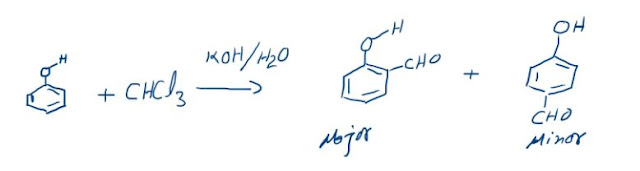## DALTON’S LAW OF PARTIAL PRESSURES

DALTON’S LAW OF PARTIAL PRESSURES:When two or more non-reacting gases are mixed in a container they tend to behave individually as there are no interactions between ideally behaving gases. The total pressure of the mixture is the sum of individual pressures of the three gases. When we say individual pressure it means the pressure that the gas would have exerted had it been the only gas in the same container. This pressure is called “Partial Pressure” of a gas. For example, If sample of a gas A is kept in a container it exerts a pressure of 2 atm, sample of gas B is kept in the same container and it exerts 3 atm then when the samples are mixed in the same container then the total pressure exerted is the sum of partial pressures that is 5 atm.

PTOTAL = PA + PB

This is called “Dalton’s Law”.
Ideal gas equation can be applied on both the gases.
PAV = nART
PBV = nBRT

Dividing,

PA/PB = nA/nB

=> PA/(PA+PB) = nA/(nA+nB)

=> PA/PTOTAL = nA/nTOTAL = XA (mole fraction of A)
Therefore the partial pressure of A,
PA = XAPTOTAL

Always remember Dalton’s Law is applicable to non-reacting gases only.

### The Reimer-Tiemann reaction: Reaction and its Mechanism

Reimer-Tiemann Reaction Phenols on reaction with chloroform in the presence of sodium hydroxide (or potassium hydroxide) solution give hydro...